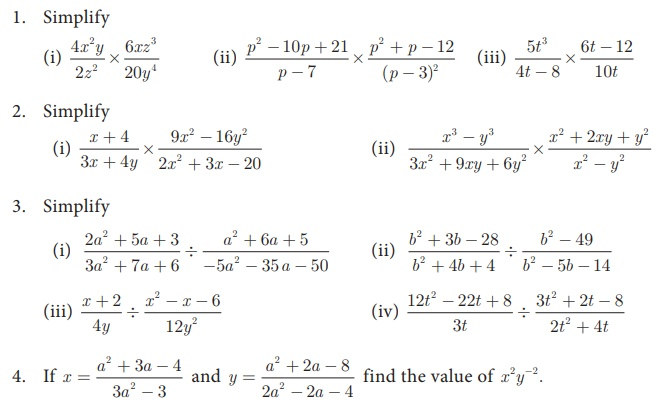Home | | Maths 10th Std | Exercise 3.5: Multiplication and Division of Rational Expressions

# Exercise 3.5: Multiplication and Division of Rational Expressions

Maths Book back answers and solution for Exercise questions - Mathematics : Algebra: Operations of Rational Expressions: Multiplication and Division of Rational Expressions: Exercise Problem Questions with Answer

### Exercise 3.55. If a polynomial p(x ) = x2  5x 14 is divided by another polynomial q (x)  we get (x7) / (x+2) , find q (x) .5. If a polynomial p(x ) = x2  5x 14 is divided by another polynomial q (x)  we get (x7) / (x+2) , find q (x) .